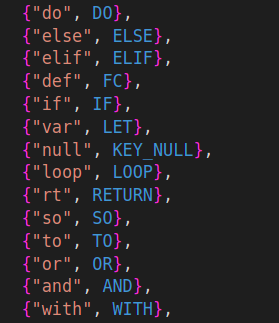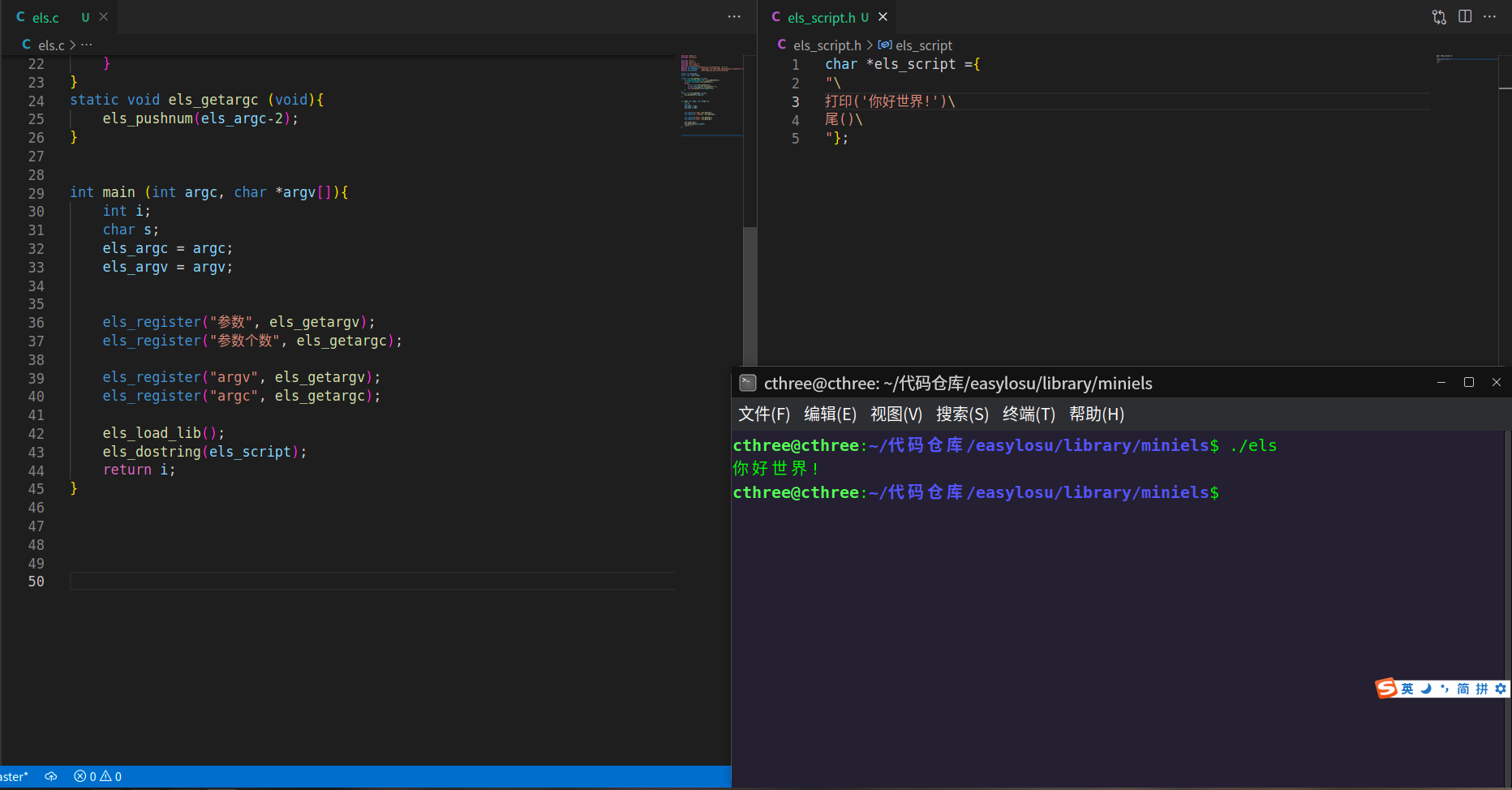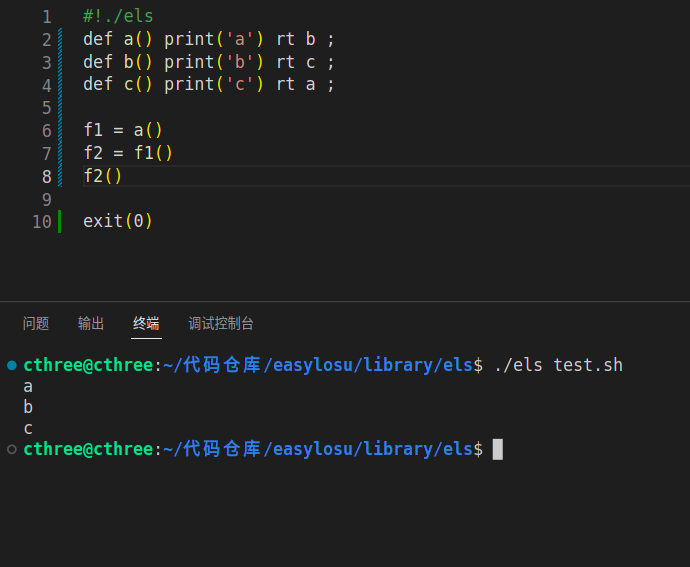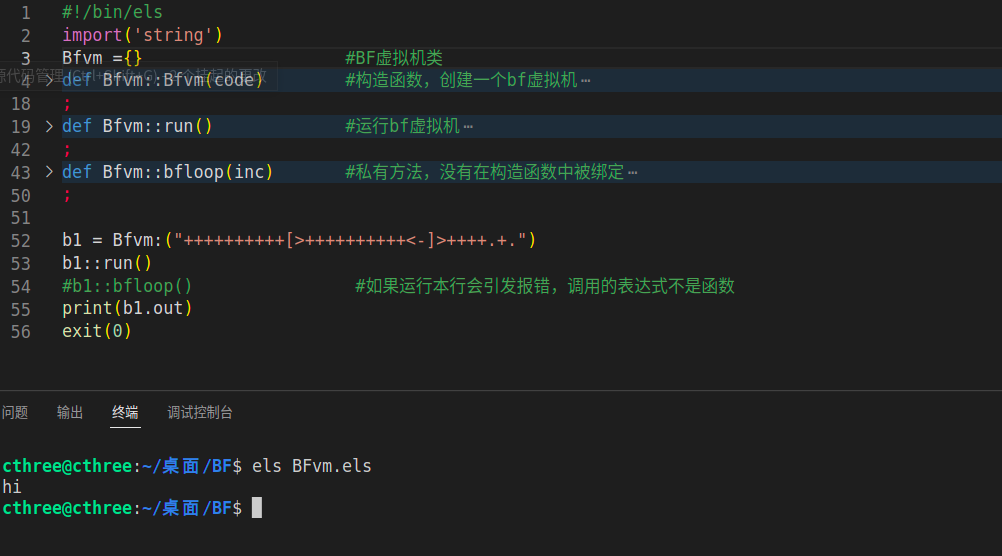# 洛书 1.5.3 发布 — 完成 1.5.1 周期内数十处反馈修订

2023-02-01 15:02:00

## 相比上一个版本，我们做了哪些更新？

### 修复大量BUG

1.  重复加载同一文件引发崩溃
2.  重复声明一个变量引发崩溃
3.  局部变量与全局变量冲突时引发崩溃
4. 逻辑运算时存在空变量引发崩溃
5. 算数运算时存在空变量或非合理类型变量时引发崩溃
6. 数值/字符 型变量 与 函数/数组/对象 类型冲突引发崩溃
7. 多层循环嵌套时局部变量引发崩溃
8. 递归时传递非值变量引发崩溃
9. 函数返回值非数值/字符 数据类型引发崩溃

### 更多新功能

#### 新增一套英文关键词，同时用户可以自由修改、添加、删除自定义的关键词。# 这是注释，英文关键词的代码
def func()
print('hello,world!')
;
func()

#### 新的语法，弱化 则/so 时/do 等二级关键词（暂时不删除，但已经不再推荐使用，后期版本会移除），统一为python风格的 :

如果 1 > 2 则
打印('你好')

打印('你好')

打印('你好')
;

if 1>2 so
print('hello')
elif 2<3 so
print('hello')
else
print('hello')
;

if 1>2 :
print('hello')
elif 2<3 :
print('hello')
else:
print('hello')
;

;

with 1<2 do
;
with 1<2 :
;

### 新的运行模式## 新数据结构与面向对象

### 新数据结构设计总则

1. 一切皆变量，函数、组、数字、字符串、类、对象本质上都是不同类型的变量
2. 变量要能够自由的修改a = 1
print(a)
def a()
print('hello')
;
a()

# 运行结果
#>1
#>hello

### 类与面向对象

# 函数 Sayhello
def Sayhello(this)
print(this.name)
;

# 创建类 Student,有一个属性 name,有一个方法sayhello
Student	={}
def Student::Student(name)
var this = {}
this.name = name
this.sayhello = Sayhello
rt this
;

# 创建类 People,有一个方法 say,与Student中sayhello方法共用一个 Sayhello()函数，Sayhello可以同时绑定给两个类
People ={}
def People::People(name)
var this = { name = name, say = Sayhello}
rt this
;

# 子类 CollegeStudent 单继承自 Student,重写sayhello方法
CollegeStudent ={}
def CollegeStudent::CollegeStudent(name)
$this = Student:(name) # 生成 this对象 this.school = 'College' # 添加 this对象的 school 属性 this.sayhello = CollegeStudent.sayhello rt this ; def CollegeStudent::sayhello() print('college:\nname:'& this.name &'\nschool:' &this.school) ; # p1对象 p1 = People:('Tom') p1::say() # s1对象 s1 = Student:('Kate') s1::sayhello() # cs1对象 cs1 = CollegeStudent:('Jane') cs1::sayhello() exit(0) ### 图灵完备性测试 感谢 凹语言 大佬提供的 样例，洛书也对BF语言的虚拟机进行了实现，作为洛书图灵完备性的一个副证。 附上相关代码： #!/bin/els 引('字符串') BF机 ={} 方法 BF机::BF机(代码)$ 此 = {}
此.输出内容  = ""
此.指令     = 代码
此.内存     = {}
此.指令地址  = 1
此.代码地址   = 0
$甲 = 0 当 甲<3000 : 甲++ 此.内存[甲]=0 ; 此.运行 = BF机.运行 回 此 ; 方法 BF机::运行() 当 此.代码地址 != 值(长度(此.指令)) : 此.代码地址 = 此.代码地址 + 1 令 甲 = 截取(此.指令,此.代码地址,1) 如果 甲==">" : 此.指令地址 = 此.指令地址 + 1 另外 甲=="<" : 此.指令地址 = 此.指令地址 - 1 另外 甲=="+" : 此.内存[此.指令地址] = 此.内存[此.指令地址] + 1 另外 甲=="-" : 此.内存[此.指令地址] = 此.内存[此.指令地址] - 1 另外 甲=="[" : 如果 此.内存[此.指令地址]==0 : BF机.循环指令(此,1) ; 另外 甲=="]" : 如果 此.内存[此.指令地址]!=0 : BF机.循环指令(此,-1) ; 另外 甲=="." : 此.输出内容 = 此.输出内容 & 字节(此.内存[此.指令地址]) ; ; ; 方法 BF机::循环指令(指令)$ 甲 = 指令
当 甲!=0 :
如果 截取(此.指令,此.代码地址+指令,1)=='[' : 甲++ ;
如果 截取(此.指令,此.代码地址+指令,1)==']' : 甲-- ;
此.代码地址 = 此.代码地址 + 指令
;
;

#!/bin/els
import('string')
Bfvm ={}                    #BF虚拟机类
def Bfvm::Bfvm(code)        #构造函数，创建一个bf虚拟机
$this = {} this.out = "" #输出内容 this.code = code #程序 this.men = {} #内存 this.pos = 1 #指令指针 this.pc = 0 #读取code的位置$ i = 0             #初始化内存空间
with i<3000 :
i++
this.men[i]=0
;
this.run    = Bfvm.run  #绑定成员函数
rt this
;
def Bfvm::run()             #运行bf虚拟机
var x
with this.pc != val(length(this.code)) :
this.pc = this.pc + 1
x = mid(this.code,this.pc,1)
if   x==">" :
this.pos = this.pos + 1
elif x=="<" :
this.pos = this.pos - 1
elif x=="+" :
this.men[this.pos] = this.men[this.pos] + 1
elif x=="-" :
this.men[this.pos] = this.men[this.pos] - 1
elif x=="[" :
if this.men[this.pos]==0 :  Bfvm.bfloop(this,1)  ;
elif x=="]" :
if this.men[this.pos]!=0 :  Bfvm.bfloop(this,-1) ;
elif x=="." :
this.out = this.out & char(this.men[this.pos])
elif x=="," :
this.men[this.pos] = ascii(input())
;
;
;
def Bfvm::bfloop(inc)       #私有方法，没有在构造函数中被绑定
\$ i = inc
with i!=0 :
if(wmid(this.code,this.pc+inc,1)=='['): i= i+1 ;
if(wmid(this.code,this.pc+inc,1)==']'): i= i-1 ;
this.pc = this.pc + inc
;
;

b1 = Bfvm:("++++++++++[>++++++++++<-]>++++.+.")
b1::run()
#b1::bfloop()                #如果运行本行会引发报错，调用的表达式不是函数
print(b1.out)
exit(0)## 1.5.3预览周期意见征集正在进行之中，您可以向相关仓库提交issues来反馈BUG及相关意见，我们将认真倾听您的每一条意见，并根据发展规划对其进行处理。2023-02-02 08:51
3

2023-02-02 10:58
2

2023-02-02 12:22
1

2023-02-01 17:54
1

### 热门文章

0 收藏

15 评论
0 收藏